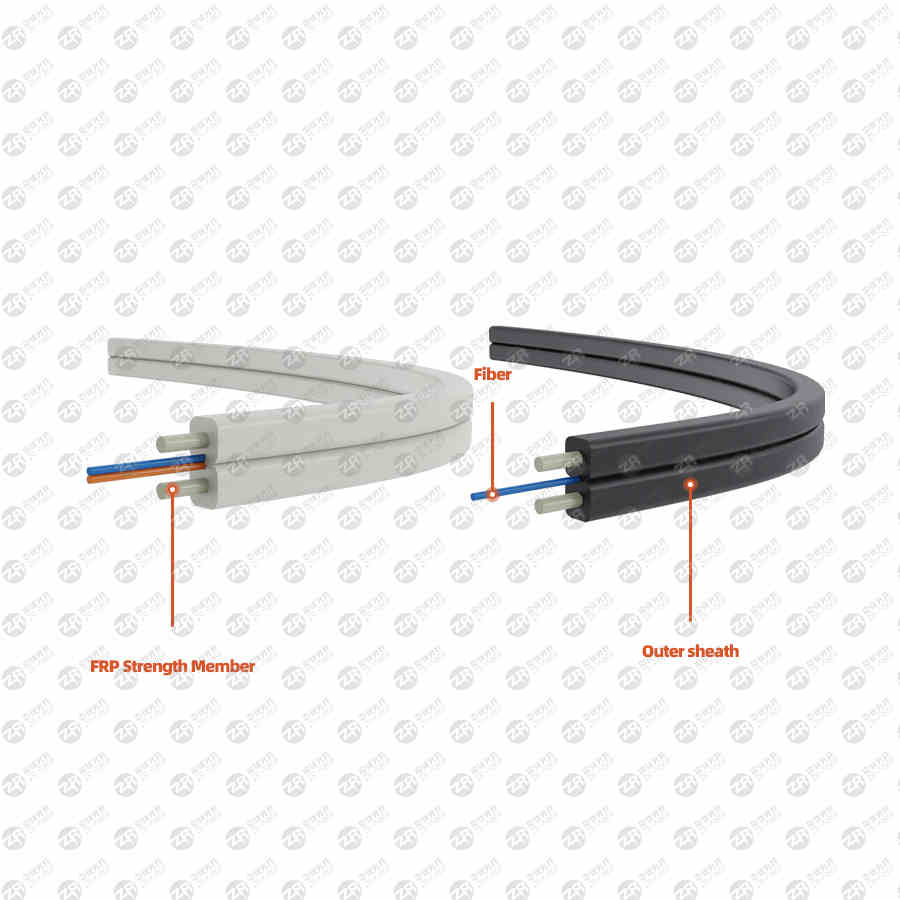# How to calculate the number of fiber splices?

2022-07-28
377

How to calculate fiber splice points? There are several ways to know the number of multi-spliced ​​cores.

1. To see how many fibers there are, multiply the number of fibers by the multiple of the fibers. For example, 12 core fibers, 12*2=24 cores, 12 cores at the beginning and 12 cores at the end;

2. Count the number of optical fiber boxes or ODF boxes, and multiply the number by the multiple of the optical fiber, such as 24-core optical fiber box (ODF), 24*2=48 cores, 24 cores at the start and 24 cores at the terminal;

The above calculation method with no optical fiber and no joint in the middle is like this. This calculation is relatively simple. The total number can be calculated by multiplying the number of optical fibers and ODF boxes by its multiple.There is also a kind of contact in the middle, which is a little troublesome. Generally, there is a phenomenon of direct fusion fiber in the middle, such as optical fiber to the home. The skin fiber of this home is fused together with the weak current fiber, and many of them will use the direct fusion method. Directly splicing in the optical fiber distribution box, no couplers and pigtails are used, only the splice tray is used to fix the optical fiber splicing point. How to calculate the number of spliced ​​fiber cores in this fiber fusion method? First, let's take a look at the composition of fiber to the home. Its composition consists of the following parts.

1. Weak current box, composed of weak current box, fiber optic disk, the fiber optic disk used here is 2 cores or 4 cores, the largest uses 8 cores, and some directly do not have fiber optic disks; row plugs; routers; switches; wired wiring frame, ONU (or light cat), etc.

2. Leather fiber, this part is generally from the weak current box to the weak current well, many of which are a summary of several floors, mainly determined according to the floor type;

3. Optical fiber, this part is from the optical fiber distribution box on the floor to the ODF cabinet in the communication room, mainly composed of optical cables; the optical cables have 6 cores, 12 cores, 24 cores, 48 ​​cores, 72 cores, 96 cores, 144 cores, 288 cores these types form fiber optic cable;

4. ODF cabinet in the computer room. The most commonly used are 576 and 720 cores;

How to calculate fiber-to-the-home fiber core? We calculate according to the above components, starting from the weak current box, the best way is to calculate according to the horizontal, vertical, and computer room parts.

1. Horizontal section. It is the weak current box to the weak current well. First of all, you need to know how many cores and sheath fibers are pulled. Then you need to know how many weak current boxes are on the floor. For example, there are 20 weak current boxes on the floor, and 20 4-core sheath fibers are placed, 20*4=80 core.

2. Vertical section. Mainly the floor optical fiber distribution box, how many sheathed fibers enter here multiplied by the number of cores of the sheathed cable; for example, 20 4-core sheathed fibers enter the optical fiber distribution box, 20*4=80 cores;

3. The computer room part. It mainly depends on how many cores of incoming optical cables are aggregated in the ODF cabinet. For example, if an ODF cabinet has 5 144-core optical cables aggregated into the cabinet, then 144*5=720 cores. Reserve a spare fiber in the box.

Through the above algorithm, the sum of the horizontal, vertical and computer room summations can be used to obtain the total number of fused fibers required for the entire project. This basically calculates the number of fibers. Having said so much, in fact, it still depends on the construction situation on site and whether the construction is carried out according to the design drawings. If the construction is carried out according to the design drawings, it can be calculated directly in the system diagram of the optical fiber to the home. Basically, how many optical fibers are counted, which is multiplied by his It can be calculated by several times. The straight-pull optical cable is multiplied by 2 times, and the jump point in the middle is multiplied by 3 times. The optical fiber fusion fiber is basically calculated in this way.

Previous OneHow to connect the fiber optic cable if the fiber optic cable is broken, can I connect it myself?
All
Next OneThe treatment method to prevent the failure of the optical module
Phone/Wechat008615211074652
Message